JOINProblem Statement
Contest: Round 3
Problem: PegJumping

### Problem Statement

You are given a square board containing N by N cells. On the board you have M types of pegs, each type has its own value for scoring purposes. A peg can move on the board by jumping over an adjacent peg and landing on an empty space. A peg can perform a sequence of jumps, one after the other. A peg will be removed from the board when another peg jumps over it. The score you get for a sequence of jumps performed by a peg is the sum of the values of the pegs removed from the board during the sequence, multiplied by the length of that sequence.

Your task is to maximize the sum of the scores gained when performing peg sequence jumps.

### Implementation Details

Your code should implement the method getMoves(int[] pegValue, String[] board). Your getMoves method will be called once and should return a String[] containing your moves.

• pegValue gives you information about each type of peg. Peg type i will add a value of pegValue[i] to the score of a sequence when it's removed from the board.
• board gives you the board containing N by N cells. Each String contains a row of the board. Any numeric character ('0'..'9') denotes the zero based type of the peg in that cell and '.' denotes an empty space.

You must return the list of peg jumps that you want to perform. Each element of your return must describe one sequence of jumps for a single peg. Each String in your return must be a space delimited string with the following format "ROW COL SEQ". ROW is the zero based row and COL the zero based column of the peg that you want to move. The top left corner of the board corresponds to row and column zero. SEQ is a list of characters that denotes the direction of each jump in the sequence. The possible directions are:

• U : Up, jumps to location (ROW-2, COL).
• D : Down, jumps to location (ROW+2, COL).
• L : Left, jumps to location (ROW, COL-2).
• R : Right, jumps to location (ROW, COL+2).

### Example

Given the following board (seed 1) and shown peg values.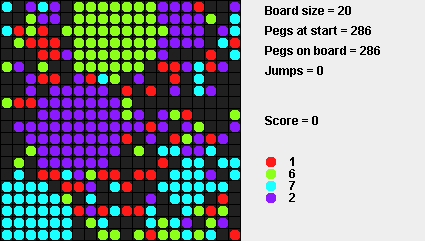Let your String[] return be {"17 11 R","11 15 LLDD"}. The first sequence will take the peg at location (17,11) and jump right, removing one peg with a total value of 7. The score for your first sequence will then be 7*1=7. The sequence is shown here: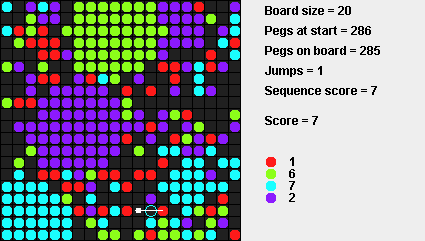Your second sequence will take the peg at location (11,15) and jump left, left, down and then down, removing 4 pegs with a total value of (1+2+6+1=10). The score for your second sequence will then be 10*4=40. The sequence is shown here: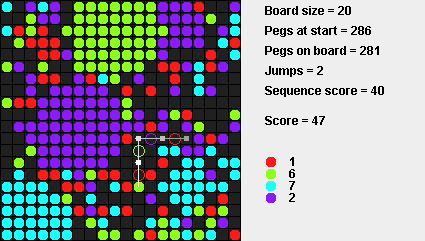If you only returned those two sequences, your total raw score for the test will be 7+40=47.

### Scoring

For each test case we will calculate your raw and normalized scores. If you were not able to produce a valid return value, then your raw score is -1 and the normalized score is 0. Otherwise, the raw score is equal to the sum of scores over all sequence jumps. The normalized score for each test is 1,000,000.0 * YOUR / BEST, where BEST is the highest score currently obtained on this test case (considering only the last submission from each competitor). Finally, your total score is equal to the arithmetic average of normalized scores on all test cases.

You can see your raw scores on each example test case by making an example submit. You can also see total scores of all competitors on provisional test set in the match standings. No other information about scores is available during the match.

### Clarifications

• Each sequence will move only one peg.
• You can use the same peg in multiple jump sequences as long as it's on the board.
• A peg will be removed from the board when another peg jumps over it.
• You don't have to remove all the pegs from the board.
• The top left corner of the board corresponds to row and column zero.

### Test Case Generation

Please look at the visualizer source code for the exact details about test case generation. Each test case is generated as follows:

• The board size N is randomly selected between 20 and 60.
• The number of peg types M is randomly selected between 1 and 10. The value of each peg type is chosen between 1 and 10.
• The fill ratio P is chosen between 0.1 and 0.9.
• A peg with a randomly selected type is placed on each cell with probability P.
• The number of blobs B on the board is chosen between 0 and 10.
• B blobs are added on the board at a randomly selected center location. The radius of each blob is chosen between 1 and N/4. The blob contains only one randomly selected peg type. The blob overwrites previously placed individual pegs.
• All values are chosen uniformly and independently, at random. Ranges are inclusive.

### Tools

An offline tester/visualizer is available here. You can use it to test/debug your solution locally. You can also check its source code for exact implementation of test case generation, simulation and score calculation.

### Definition

 Class: PegJumping Method: getMoves Parameters: int[], String[] Returns: String[] Method signature: String[] getMoves(int[] pegValue, String[] board) (be sure your method is public)

### Notes

-The time limit is 15 seconds per test case (this includes only the time spent in your code). The memory limit is 1024 megabytes.
-There is no explicit code size limit. The implicit source code size limit is around 1 MB (it is not advisable to submit codes of size close to that or larger). Once your code is compiled, the binary size should not exceed 1 MB.
-The compilation time limit is 30 seconds. You can find information about compilers that we use and compilation options here.
-There are 10 example test cases and 100 full submission (provisional) test cases.

### Examples

0)

```Seed = 1
```1)

```Seed = 2
```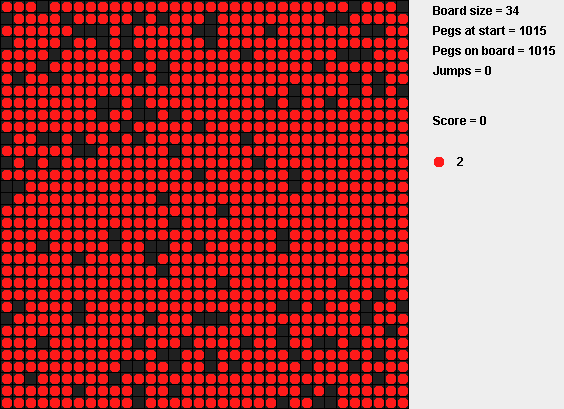2)

```Seed = 3
```3)

```Seed = 4
```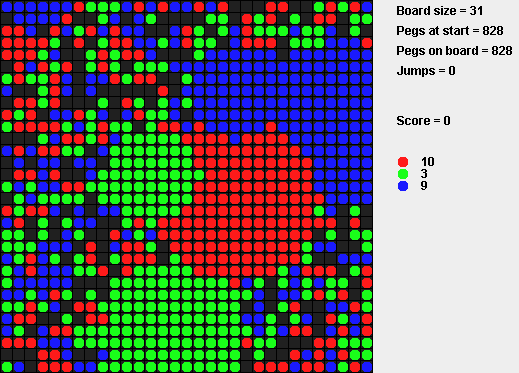4)

```Seed = 5
```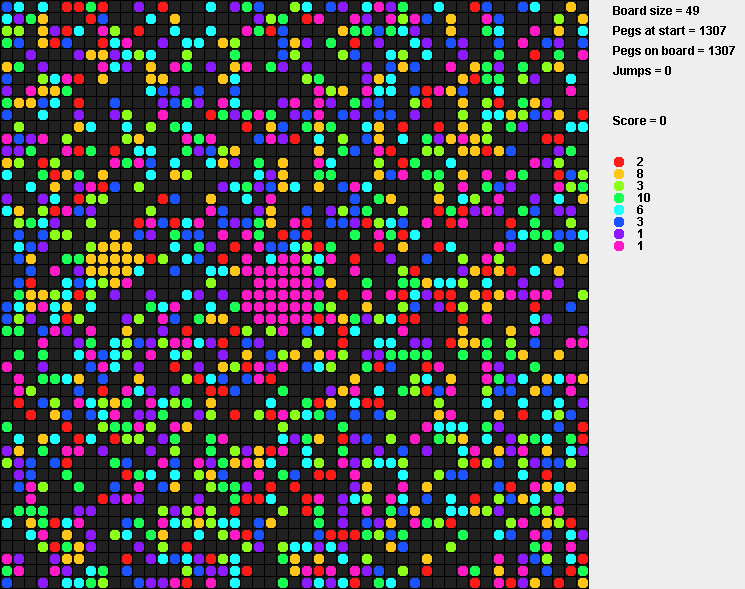5)

```Seed = 6
```
6)

```Seed = 7
```
7)

```Seed = 8
```
8)

```Seed = 9
```
9)

```Seed = 10
```

This problem statement is the exclusive and proprietary property of TopCoder, Inc. Any unauthorized use or reproduction of this information without the prior written consent of TopCoder, Inc. is strictly prohibited. (c)2010, TopCoder, Inc. All rights reserved.